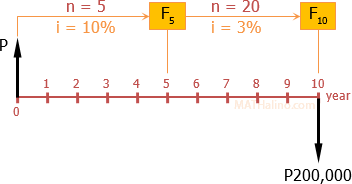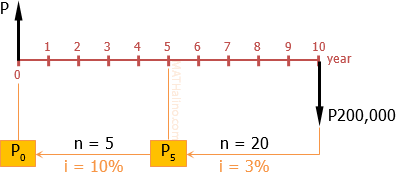# MIGRATED: interest and compound: A man wishes his son to receive P200,000 ten years from now

5 posts / 0 new
Sydney SalesMIGRATED: interest and compound: A man wishes his son to receive P200,000 ten years from now

A man wishes his son to receive P200 000 ten years from now. What amount should he invest now if it will earn interest of 10% compounded annually during the first 5 years and 12% compounded quarterly during the next 5 years.

Tags:
Jhun VertSolution 1
$F = P(1 + i)^n$$F_5 = P(1 + 0.10)^5$

$F_5 = 1.10^5P$

$F_{10} = F_5(1 + 0.03)^{20}$

$F_{10} = 1.10^5P(1.03^{20})$

$F_{10} = (1.10^5)(1.03^{20})P$

$F_{10} = 20\,000$

$(1.10^5)(1.03^{20})P = 20\,000$

$P = \text{P}6,875.78$       answer

edgineer (guest)

Wrong answer. The future worth is 200,000 not 20,000

ggneer (guest)

F = 200,000. Therfore P=68757.81

Jhun VertSolution 2
$P = \dfrac{F}{(1 + i)^n}$$P_5 = \dfrac{20\,000}{(1 + 0.03)^{20}}$

$P_5 = \text{P}11,073.52$

$P_0 = \dfrac{P_5}{(1 + 0.10)^5}$

$P_0 = \dfrac{11,073.52}{1.10^5}$

$P_0 = \text{P}6,875.78$

$P = P_0$

$P = \text{P}6,875.78$       answer

• Mathematics inside the configured delimiters is rendered by MathJax. The default math delimiters are $$...$$ and $...$ for displayed mathematics, and $...$ and $...$ for in-line mathematics.